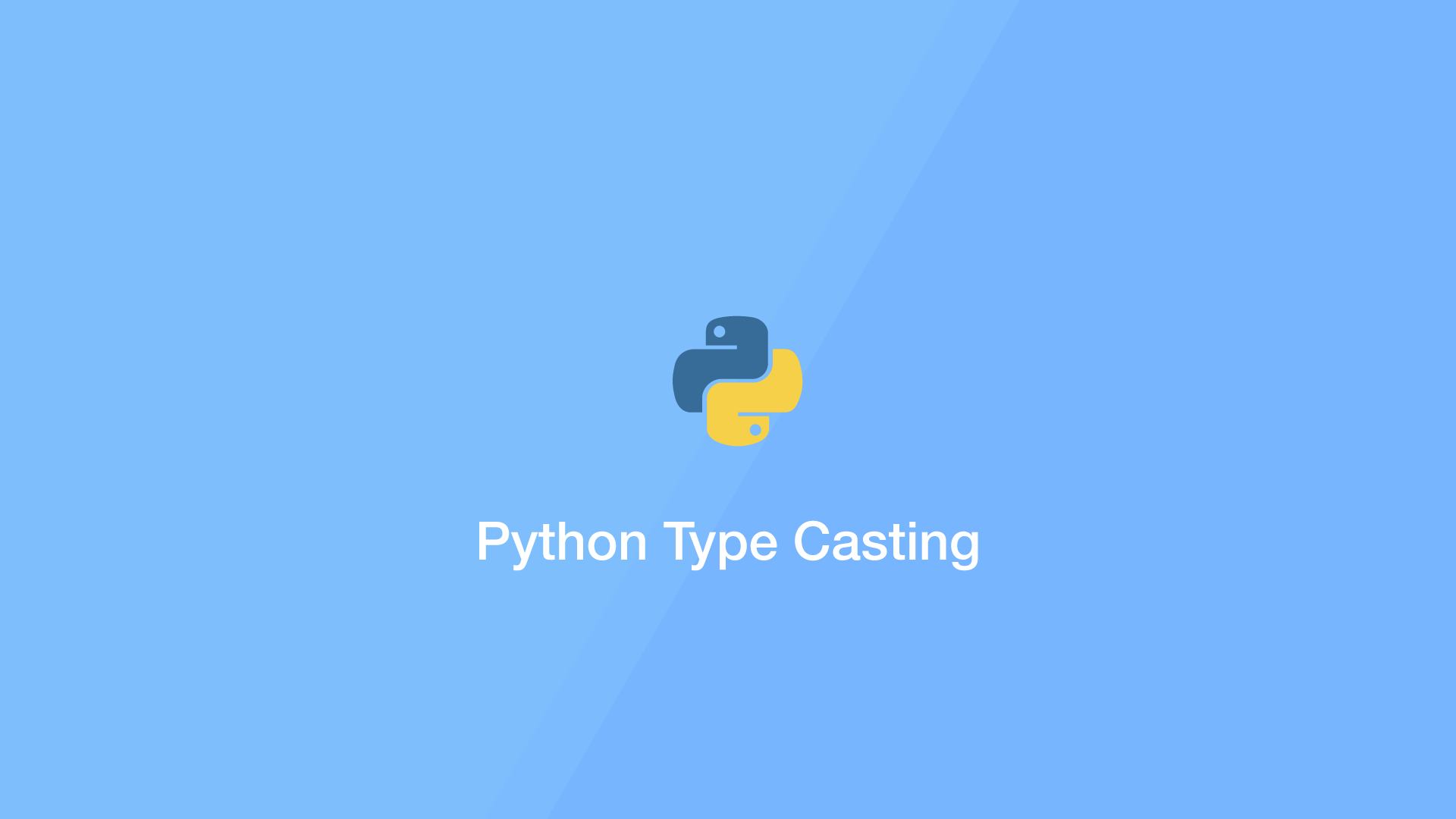# Python Type Conversion and Type CastingTypecasting in Python is the process of converting an item with a particular data type to another data type.

In Python we can use the built-in `int()`, `float()` and `str()` functions to convert the data type of any item to an integer, floating-point number or string provided the literal contains appropriate values.

## Type Cast int to float

To cast an int to a float, use the Python `float()` function and pass the value to change as the first argument:

``````i = 92

f = float(i)

print(f)
print(type(f))
``````
``````92.0
<class 'float'>
``````

As you can see from the output, the number is now cast as `class 'float'`. It is important to note that the `float()` function does not modify the original variable.

## Type Cast float to int

To cast an integer, use the `int()` function and pass the floating-point number to change as the first argument:

``````f = 92.1

i = int(f)

print(i)
print(type(i))
``````
``````92
<class 'int'>
``````

Note – no rounding is done with the `int()` function, the decimal place is simply removed.

## Type Cast string to int and float

Strings can also be changed to floats and integers like this:

``````s = '92.1'

f = float(s)
i = int(f)

print(i)
print(type(i))

print(f)
print(type(f))
``````
``````92
<class 'int'>
92.1
<class 'float'>
``````

If the string contains a float, it must be cast as a float before casting to an integer or you will get a `ValueError`.

#### Related Tutorials### How to Concatenate Strings in Python

June 22, 2021### Python Print Formatting

September 24, 2020### How to Parse JSON Data in Python (Read and Write)

September 18, 2020### How to Read CSV Files in Python

October 02, 2020### How to Use Argparse in Python

September 25, 2020### How to use Python datetime to Create and use Dates and Times

September 24, 2020